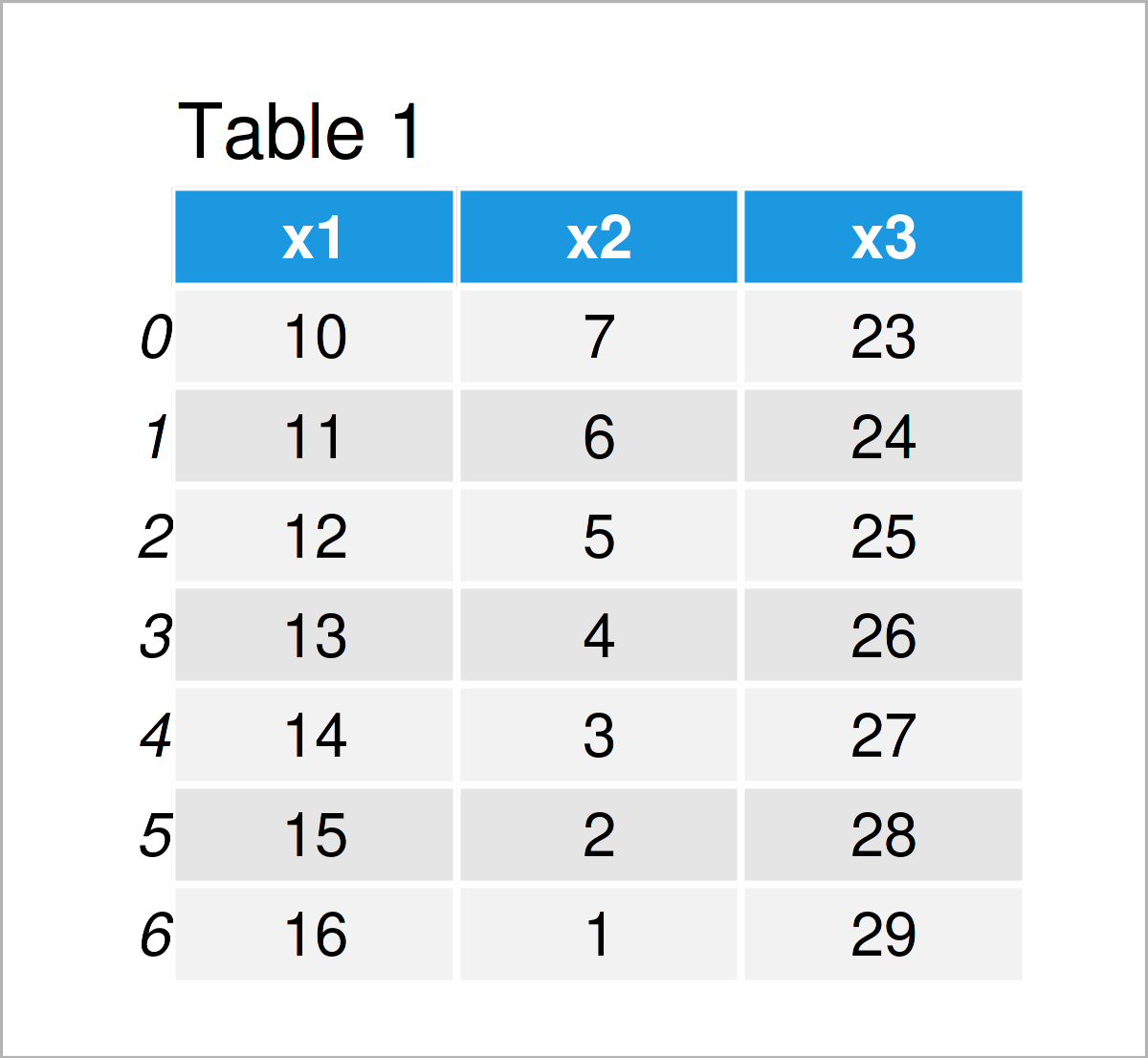# Convert pandas DataFrame to List in Python (3 Examples)

This article illustrates how to convert values in a pandas DataFrame to a list object in the Python programming language.

The content is structured as follows:

Let’s start right away:

## Example Data & Software Libraries

We first need to import the pandas library to Python, if we want to use the functions that are contained in the library:

`import pandas as pd                         # Import pandas`

The pandas DataFrame below will be used as a basis for this Python tutorial:

```data = pd.DataFrame({'x1':range(10, 17),    # Create pandas DataFrame
'x2':range(7, 0, - 1),
'x3':range(23, 30)})
print(data)                                 # Print pandas DataFrame```Have a look at the table that has been returned after executing the previously shown Python syntax. It shows that our exemplifying data contains seven rows and three columns.

## Example 1: Extract pandas DataFrame Column as List

In Example 1, I’ll demonstrate how to convert a specific column of a pandas DataFrame to a list object in Python.

For this task, we can use the tolist function as shown below:

```list1 = data['x1'].tolist()                 # Select column
print(list1)                                # Print list
# [10, 11, 12, 13, 14, 15, 16]```

Have a look at the previous console output: It shows that we have created a new list object containing the elements of the first column x1.

## Example 2: Extract pandas DataFrame Row as List

In this example, I’ll show how to select a certain row of a pandas DataFrame and transform it to a list object.

This time, we have to extract the values using the .loc attribute. These values can then be converted to a list object using the tolist function:

```list2 = data.loc[].values.tolist()       # Select row
print(list2)                                # Print list
# [[10, 7, 23]]```

## Example 3: Convert Entire pandas DataFrame to List

In this example, I’ll demonstrate how to convert all elements in the rows and columns of a pandas DataFrame to a list object.

For this, we have to use the .values attribute and the tolist function as shown below:

```list3 = data.values.tolist()                # Convert all values
print(list3)                                # Print list
# [[10, 7, 23], [11, 6, 24], [12, 5, 25], [13, 4, 26], [14, 3, 27], [15, 2, 28], [16, 1, 29]]```

## Video & Further Resources

Do you need more info on the Python programming syntax of this tutorial? Then you could have a look at the following video on my YouTube channel. I’m explaining the topics of this tutorial in the video.

Please accept YouTube cookies to play this video. By accepting you will be accessing content from YouTube, a service provided by an external third party.If you accept this notice, your choice will be saved and the page will refresh.

In addition, you could have a look at some of the related tutorials on my website. You can find a selection of articles that are related to the transformation of values in a pandas DataFrame to a list object below:

In summary: At this point of the tutorial you should have learned how to convert a pandas DataFrame to a list object in the Python programming language. Please let me know in the comments, in case you have further questions.

Subscribe to the Statistics Globe Newsletter

•Raveena
March 9, 2023 9:55 pm

I need help!

All the data is in 1 cell. How can I convert it into rows and columns and save it as csv?

•March 15, 2023 11:46 am

Hello Raveena,

If you want to convert a pandas DataFrame to a csv file, you use the pandas.DataFrame.to_csv() method, you can check our tutorial https://statisticsglobe.com/write-pandas-dataframe-csv-file-pythonWrite pandas DataFrame to CSV File in Python (4 Examples).

In order to reformat the data which is entirely stored in a single cell, you need to initialize more columns and split the data to them, this requires different approaches depending on how the data is stored in a single cell, can you describe the format of the data further?

If you have further questions, please let us know.

Best,
Ömer

•Raveena
April 12, 2023 5:02 pm

Hi Omer,
Thank you very much for your help! I have learned a lot from this. Now the problem is, I can save the data in csv file and in different columns, but all the different lists come in one column. Is it possible that I can share the csv file. Will you be able to help me with this? Unfortunately, I will not be able to share python file, but I can share the csv file.
•April 17, 2023 6:24 pm